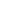# Free CBSE Class 10 Mathematics UNIT IV: Geometry Circles Worksheets

Download free printable Circles Worksheets to practice. With thousands of questions available, you can generate as many Circles Worksheets as you want.

## Sample CBSE Class 10 Mathematics UNIT IV: Geometry Circles Worksheet Questions

1.

Point is also a circle.

1. True 2. False
2.

In the given figure, PA and PB are two tangents drawn from an external point P to a circle with centre C and radius 4cm. If PA⊥ PB, then the length of each tangent is____1.

8 cm

2.

5 cm

3.

2 cm

4.

4 cm

3.

In the given figure, a circle is inscribed in a triangle PQR with PQ = 10 cm, QR = 8 cm and PR = 12 cm. Find the length of RN?1.

5 cm

2.

3 cm

3.

2 cm

4.

4 cm

4.

In the given figure, two circles with centres A and B touch each other externally at k. The length of PQ is_____1.

18 cm

2.

20 cm

3.

27 cm

4.

24 cm

5.

In the given figure, PA and PB are two tangents drawn to a circle with centre O and radius r. If OP = 2r, then APB  is___1.

Isosceles

2.

Scalene

3.

Equilateral

4.

None of these

6.

In given figure,  ABC is right angled at B. AB = 6 cm, BC = 8 cm. Find the radius r of the circle inscribed.1.

1.5 cm

2.

3 cm

3.

2 cm

4.

4 cm

7.

In the given, if PA and PB are tangents to the circle with centre O such that , then is equal to ____1.

2.

3.

4.

8.

In the given figure, AP, AQ and BC are tangents to circle.  If AB = 5 cm, AC = 6 cm and BC = 4 cm, then the length of AP is____1.

15 cm

2.

12 cm

3.

10 cm

4.

7.5 cm

9.

In given figure, a circle touches the side QR of  PQR at A and sides PQ and PR on producing at S and T respectively. If PS = 7 cm, find the perimeter of PQR.1.

12 cm

2.

14 cm

3.

7 cm

4.

21 cm

10.

In the given figure, AB is a chord of the circle and AOC is its diameter such that . If AT is the tangent to the circle at the point A, then  is equal to_____1.

2.

3.

4.

Worksheets by UrbanPro

Our worksheets are designed to help students explore various topics, practice skills and enrich their subject knowledge, to improve their academic performance. Designed by Experts who have extensive experience and expertise in teaching a subject, these worksheets will improve your child's problem-solving skills and subject knowledge in a fun and interactive manner.
Check out our free customized worksheets across school boards, grades, subjects and levels of subject knowledge. You can download, print and share these worksheets with anyone, anywhere, anytime!

Get a custom worksheet to practice!

Select your topic & see the magic.

subjectSelect Chapter(s)

Chapters & Subtopics